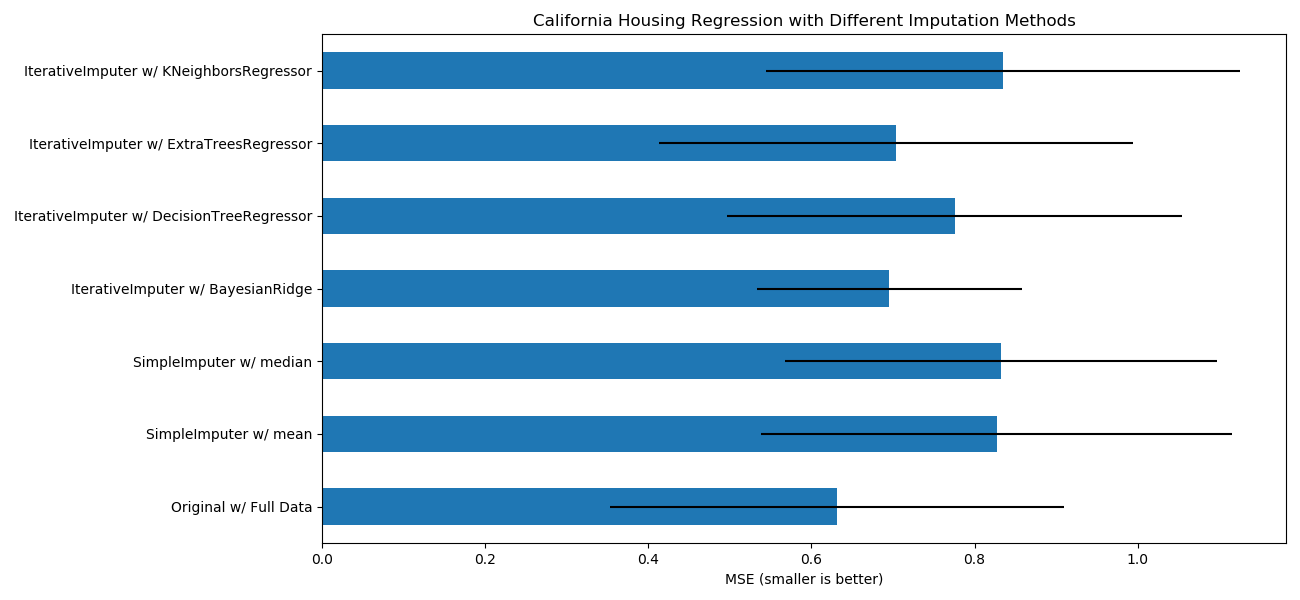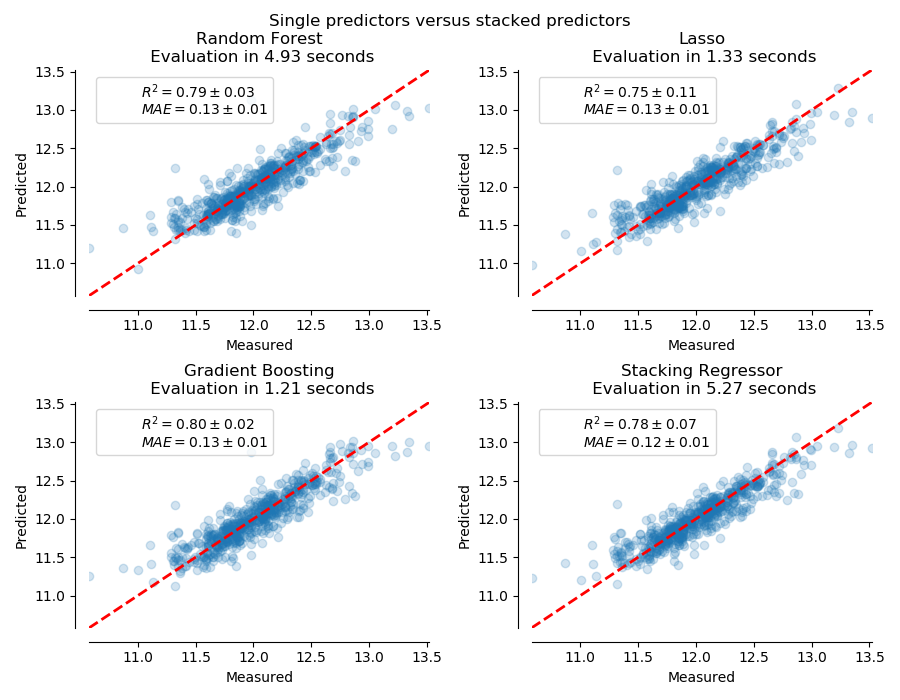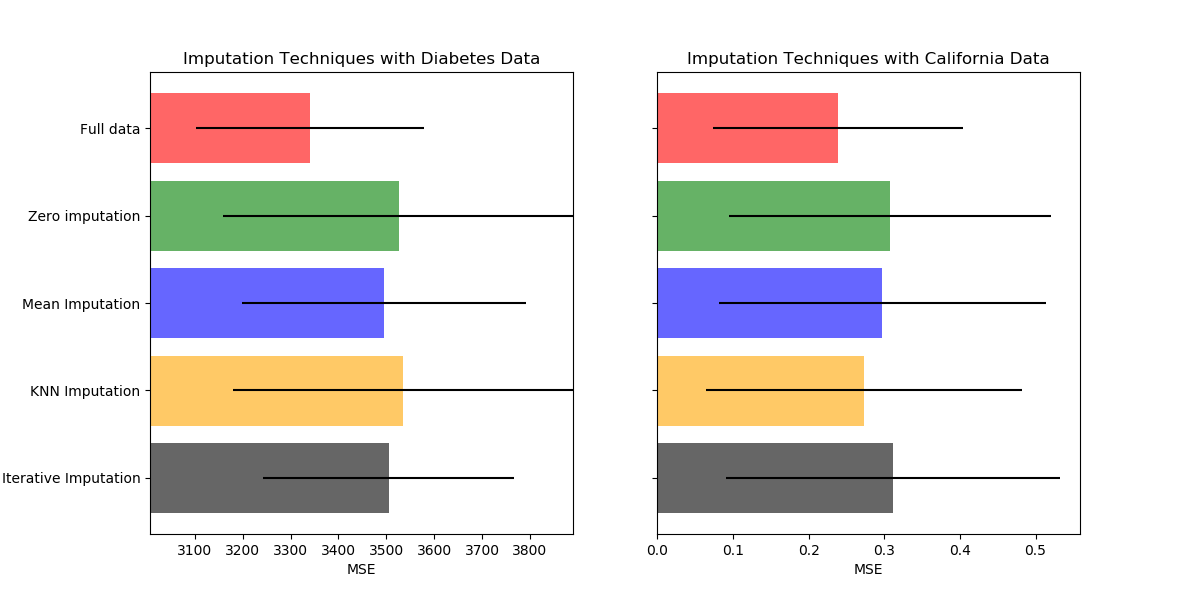# sklearn.ensemble.RandomForestRegressor¶

class sklearn.ensemble.RandomForestRegressor(n_estimators=100, *, criterion='mse', max_depth=None, min_samples_split=2, min_samples_leaf=1, min_weight_fraction_leaf=0.0, max_features='auto', max_leaf_nodes=None, min_impurity_decrease=0.0, min_impurity_split=None, bootstrap=True, oob_score=False, n_jobs=None, random_state=None, verbose=0, warm_start=False, ccp_alpha=0.0, max_samples=None)

[源码]

n_estimators int, default=100

criterion {“mse”, “mae”}, default=”mse”

max_depth int, default=None

min_samples_split int or float, default=2

- 如果为int，则认为min_samples_split是最小值。
- 如果为float，min_samples_split则为分数， 是每个拆分的最小样本数。
ceil(min_samples_split * n_samples)

min_samples_leaf int or float, default=1

- 如果为int，则认为min_samples_leaf是最小值。
- 如果为float，min_samples_leaf则为分数, 是每个节点的最小样本数。
ceil(min_samples_leaf * n_samples)

min_weight_fraction_leaf float, default=0.0

max_features {“auto”, “sqrt”, “log2”}, int or float, default=”auto”

- 如果为int，则max_features在每个分割处考虑特征。
- 如果为float，max_features则为小数，并 在每次拆分时考虑要素。
- 如果为auto，则为max_features=sqrt(n_features)
- 如果是sqrt，则max_features=sqrt(n_features)
- 如果为log2，则为max_features=log2(n_features)
- 如果为None，则max_features=n_features

max_leaf_nodes int, default=None
max_leaf_nodes以最好的方式进行“种树”。杂质的相对减少的节点被当作最佳节点。如果为None，则叶节点数不受限制。
min_impurity_decrease float, default=0.0

N_t / N * (impurity - N_t_R / N_t * right_impurity - N_t_L / N_t * left_impurity)

NN_tN_t_R并且N_t_L都指的是加权和，如果sample_weight获得通过。

min_impurity_split float, default=None

- 从版本0.19min_impurity_split开始不推荐使用：在版本0.19中不再推荐使用 min_impurity_decrease。的默认值 min_impurity_split在0.23中从1e-7更改为0，并将在0.25中删除。使用min_impurity_decrease代替。
bootstrap bool, default=False

oob_score bool, default=False

n_jobs int, default=None

random_state int, RandomState, default=None

- 构建树木时使用的示例的引导程序(如果bootstrap=True)
- 在每个节点上寻找最佳分割时要考虑的特征采样（如果max_features < n_features)
- 绘制每个max_features的分割

verbose int, default=0

warm_start bool, default=False

ccp_alpha non-negative float, default=0.0

0.22版中的新功能。
max_samples int or float, default=None

- 如果为None(默认)，则绘制X.shape样本。
- 如果为int，则绘制 max_samples 样本。
- 如果为float, 则绘制 max_samples * X.shape样本。因此，max_samples应该在区间(0,1)内。
0.22版本新增功能

base_estimator_ DecisionTreeRegressor

estimators_ list of DecisionTreeRegressor

feature_importances_ ndarray of shape (n_features,)

n_features_ int

n_outputs_ int

oob_score_ float

oob_prediction_ ndarray of shape (n_samples,)

DecisionTreeRegressor, ExtraTreesRegressor

1. Breiman, “Random Forests”, Machine Learning, 45(1), 5-32, 2001.

2. P. Geurts, D. Ernst., and L. Wehenkel, “Extremely randomized trees”, Machine Learning, 63(1), 3-42, 2006.

>>> from sklearn.ensemble import RandomForestRegressor>>> from sklearn.datasets import make_regression>>> X, y = make_regression(n_features=4, n_informative=2,...                        random_state=0, shuffle=False)>>> regr = RandomForestRegressor(max_depth=2, random_state=0)>>> regr.fit(X, y)RandomForestRegressor(...)>>> print(regr.predict([[0, 0, 0, 0]]))[-8.32987858]

apply(X) 将森林中的树应用于X，返回叶子索引。
decision_path(X) 返回森林中的决策路径。
fit(X, y[, sample_weight]) 从训练集(X, y)构建一个树的森林。
get_params([deep]) 获取此估计器的参数。
predict(X) 为X预测回归目标。
score(X, y[, sample_weight]) 返回预测的决定系数R^2
set_params(**params) 设置此估算器的参数。
__init__(n_estimators=100, *, criterion='mse', max_depth=None, min_samples_split=2, min_samples_leaf=1, min_weight_fraction_leaf=0.0, max_features='auto', max_leaf_nodes=None, min_impurity_decrease=0.0, min_impurity_split=None, bootstrap=True, oob_score=False, n_jobs=None, random_state=None, verbose=0, warm_start=False, ccp_alpha=0.0, max_samples=None)

[源码]

apply(X)

[源码]

X {array-like, sparse matrix} of shape (n_samples, n_features)

X_leaves ndarray of shape (n_samples, n_estimators)

decision_path(X)

[源码]

X {array-like, sparse matrix} of shape (n_samples, n_features)

indicator sparse matrix of shape (n_samples, n_nodes)

n_nodes_ptr ndarray of shape (n_estimators + 1,)

property feature_importances_

feature_importances_ ndarray of shape (n_features,)

fit(X, y, sample_weight = None)

[源码]

X {array-like, sparse matrix} of shape (n_samples, n_features)

y array-like of shape (n_samples,) or (n_samples, n_outputs)

sample_weight array-like of shape (n_samples,), default=None

self object
get_params(deep=True)

[源码]

deep bool, default=True

params mapping of string to any

predict(X)

[源码]

X {array-like, sparse matrix} of shape (n_samples, n_features)

y ndarray of shape (n_samples,) or (n_samples, n_outputs)

score(X, y, sample_weight=None)

[源码]

X array-like of shape (n_samples, n_features)

y array-like of shape (n_samples,) or (n_samples, n_outputs)
X的正确值。
sample_weight array-like of shape (n_samples,), default=None

score float
self.predict(X) 关于y的准确率。

set_params(**params)

[源码]

**params dict

self object

## sklearn.ensemble.RandomForestRegressor使用示例¶